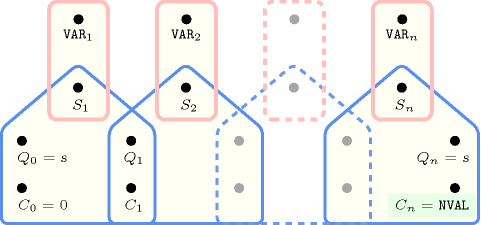## 5.173. group_skip_isolated_item

Origin

Derived from $\mathrm{𝚐𝚛𝚘𝚞𝚙}$.

Constraint

$\mathrm{𝚐𝚛𝚘𝚞𝚙}_\mathrm{𝚜𝚔𝚒𝚙}_\mathrm{𝚒𝚜𝚘𝚕𝚊𝚝𝚎𝚍}_\mathrm{𝚒𝚝𝚎𝚖}\left(\begin{array}{c}\mathrm{𝙽𝙶𝚁𝙾𝚄𝙿},\hfill \\ \mathrm{𝙼𝙸𝙽}_\mathrm{𝚂𝙸𝚉𝙴},\hfill \\ \mathrm{𝙼𝙰𝚇}_\mathrm{𝚂𝙸𝚉𝙴},\hfill \\ \mathrm{𝙽𝚅𝙰𝙻},\hfill \\ \mathrm{𝚅𝙰𝚁𝙸𝙰𝙱𝙻𝙴𝚂},\hfill \\ \mathrm{𝚅𝙰𝙻𝚄𝙴𝚂}\hfill \end{array}\right)$

Arguments
 $\mathrm{𝙽𝙶𝚁𝙾𝚄𝙿}$ $\mathrm{𝚍𝚟𝚊𝚛}$ $\mathrm{𝙼𝙸𝙽}_\mathrm{𝚂𝙸𝚉𝙴}$ $\mathrm{𝚍𝚟𝚊𝚛}$ $\mathrm{𝙼𝙰𝚇}_\mathrm{𝚂𝙸𝚉𝙴}$ $\mathrm{𝚍𝚟𝚊𝚛}$ $\mathrm{𝙽𝚅𝙰𝙻}$ $\mathrm{𝚍𝚟𝚊𝚛}$ $\mathrm{𝚅𝙰𝚁𝙸𝙰𝙱𝙻𝙴𝚂}$ $\mathrm{𝚌𝚘𝚕𝚕𝚎𝚌𝚝𝚒𝚘𝚗}\left(\mathrm{𝚟𝚊𝚛}-\mathrm{𝚍𝚟𝚊𝚛}\right)$ $\mathrm{𝚅𝙰𝙻𝚄𝙴𝚂}$ $\mathrm{𝚌𝚘𝚕𝚕𝚎𝚌𝚝𝚒𝚘𝚗}\left(\mathrm{𝚟𝚊𝚕}-\mathrm{𝚒𝚗𝚝}\right)$
Restrictions
 $\mathrm{𝙽𝙶𝚁𝙾𝚄𝙿}\ge 0$ $3*\mathrm{𝙽𝙶𝚁𝙾𝚄𝙿}\le |\mathrm{𝚅𝙰𝚁𝙸𝙰𝙱𝙻𝙴𝚂}|+1$ $\mathrm{𝙼𝙸𝙽}_\mathrm{𝚂𝙸𝚉𝙴}\ge 0$ $\mathrm{𝙼𝙸𝙽}_\mathrm{𝚂𝙸𝚉𝙴}\ne 1$ $\mathrm{𝙼𝙰𝚇}_\mathrm{𝚂𝙸𝚉𝙴}\ge \mathrm{𝙼𝙸𝙽}_\mathrm{𝚂𝙸𝚉𝙴}$ $\mathrm{𝙽𝚅𝙰𝙻}\ge \mathrm{𝙼𝙰𝚇}_\mathrm{𝚂𝙸𝚉𝙴}$ $\mathrm{𝙽𝚅𝙰𝙻}\ge \mathrm{𝙽𝙶𝚁𝙾𝚄𝙿}$ $\mathrm{𝙽𝚅𝙰𝙻}\le |\mathrm{𝚅𝙰𝚁𝙸𝙰𝙱𝙻𝙴𝚂}|$ $\mathrm{𝚛𝚎𝚚𝚞𝚒𝚛𝚎𝚍}$$\left(\mathrm{𝚅𝙰𝚁𝙸𝙰𝙱𝙻𝙴𝚂},\mathrm{𝚟𝚊𝚛}\right)$ $\mathrm{𝚛𝚎𝚚𝚞𝚒𝚛𝚎𝚍}$$\left(\mathrm{𝚅𝙰𝙻𝚄𝙴𝚂},\mathrm{𝚟𝚊𝚕}\right)$ $\mathrm{𝚍𝚒𝚜𝚝𝚒𝚗𝚌𝚝}$$\left(\mathrm{𝚅𝙰𝙻𝚄𝙴𝚂},\mathrm{𝚟𝚊𝚕}\right)$
Purpose

Let $n$ be the number of variables of the collection $\mathrm{𝚅𝙰𝚁𝙸𝙰𝙱𝙻𝙴𝚂}$. Let ${X}_{i},{X}_{i+1},\cdots ,{X}_{j}$ $\left(1\le i be consecutive variables of the collection of variables $\mathrm{𝚅𝙰𝚁𝙸𝙰𝙱𝙻𝙴𝚂}$ such that the following conditions apply:

• All variables ${X}_{i},\cdots ,{X}_{j}$ take their value in the set of values $\mathrm{𝚅𝙰𝙻𝚄𝙴𝚂}$,

• $i=1$ or ${X}_{i-1}$ does not take a value in $\mathrm{𝚅𝙰𝙻𝚄𝙴𝚂}$,

• $j=n$ or ${X}_{j+1}$ does not take a value in $\mathrm{𝚅𝙰𝙻𝚄𝙴𝚂}$.

We call such a set of variables a group. The constraint $\mathrm{𝚐𝚛𝚘𝚞𝚙}_\mathrm{𝚜𝚔𝚒𝚙}_\mathrm{𝚒𝚜𝚘𝚕𝚊𝚝𝚎𝚍}_\mathrm{𝚒𝚝𝚎𝚖}$ is true if all the following conditions hold:

• There are exactly $\mathrm{𝙽𝙶𝚁𝙾𝚄𝙿}$ groups of variables,

• The number of variables of the smallest group is $\mathrm{𝙼𝙸𝙽}_\mathrm{𝚂𝙸𝚉𝙴}$,

• The number of variables of the largest group is $\mathrm{𝙼𝙰𝚇}_\mathrm{𝚂𝙸𝚉𝙴}$,

• The number of variables that take their value in the set of values $\mathrm{𝚅𝙰𝙻𝚄𝙴𝚂}$ is equal to $\mathrm{𝙽𝚅𝙰𝙻}$.

Example
$\left(1,2,2,3,〈2,8,1,7,4,5,1,1,1〉,〈0,2,4,6,8〉\right)$

Given the fact that groups are formed by even values in $\left\{0,2,4,6,8\right\}$ (i.e., values expressed by the $\mathrm{𝚅𝙰𝙻𝚄𝙴𝚂}$ collection), and the fact that isolated even values are ignored, the $\mathrm{𝚐𝚛𝚘𝚞𝚙}_\mathrm{𝚜𝚔𝚒𝚙}_\mathrm{𝚒𝚜𝚘𝚕𝚊𝚝𝚎𝚍}_\mathrm{𝚒𝚝𝚎𝚖}$ constraint holds since:

• Its first argument, $\mathrm{𝙽𝙶𝚁𝙾𝚄𝙿}$, is set to value 1 since the sequence $281745111$ contains only one group of even values involving more than one even value (i.e., group $28$).

• Its second and third arguments, $\mathrm{𝙼𝙸𝙽}_\mathrm{𝚂𝙸𝚉𝙴}$ and $\mathrm{𝙼𝙰𝚇}_\mathrm{𝚂𝙸𝚉𝙴}$, are both set to 2 since the only group of even values with more than one even value involves two values (i.e., group $28$).

• The fourth argument, $\mathrm{𝙽𝚅𝙰𝙻}$, is fixed to 2 since it corresponds to the total number of even values belonging to groups involving more than one even value (i.e., value 4 is discarded since it is an isolated even value of the sequence $281745111$).

Typical
 $\mathrm{𝙽𝙶𝚁𝙾𝚄𝙿}>0$ $\mathrm{𝙼𝙸𝙽}_\mathrm{𝚂𝙸𝚉𝙴}>0$ $\mathrm{𝙽𝚅𝙰𝙻}>\mathrm{𝙼𝙰𝚇}_\mathrm{𝚂𝙸𝚉𝙴}$ $\mathrm{𝙽𝚅𝙰𝙻}>\mathrm{𝙽𝙶𝚁𝙾𝚄𝙿}$ $\mathrm{𝙽𝚅𝙰𝙻}<|\mathrm{𝚅𝙰𝚁𝙸𝙰𝙱𝙻𝙴𝚂}|$ $|\mathrm{𝚅𝙰𝚁𝙸𝙰𝙱𝙻𝙴𝚂}|>1$ $\mathrm{𝚛𝚊𝚗𝚐𝚎}$$\left(\mathrm{𝚅𝙰𝚁𝙸𝙰𝙱𝙻𝙴𝚂}.\mathrm{𝚟𝚊𝚛}\right)>1$ $|\mathrm{𝚅𝙰𝙻𝚄𝙴𝚂}|>0$ $|\mathrm{𝚅𝙰𝚁𝙸𝙰𝙱𝙻𝙴𝚂}|>|\mathrm{𝚅𝙰𝙻𝚄𝙴𝚂}|$
Symmetries
• Items of $\mathrm{𝚅𝙰𝚁𝙸𝙰𝙱𝙻𝙴𝚂}$ can be reversed.

• Items of $\mathrm{𝚅𝙰𝙻𝚄𝙴𝚂}$ are permutable.

• An occurrence of a value of $\mathrm{𝚅𝙰𝚁𝙸𝙰𝙱𝙻𝙴𝚂}.\mathrm{𝚟𝚊𝚛}$ that belongs to $\mathrm{𝚅𝙰𝙻𝚄𝙴𝚂}.\mathrm{𝚟𝚊𝚕}$ (resp. does not belong to $\mathrm{𝚅𝙰𝙻𝚄𝙴𝚂}.\mathrm{𝚟𝚊𝚕}$) can be replaced by any other value in $\mathrm{𝚅𝙰𝙻𝚄𝙴𝚂}.\mathrm{𝚟𝚊𝚕}$ (resp. not in $\mathrm{𝚅𝙰𝙻𝚄𝙴𝚂}.\mathrm{𝚟𝚊𝚕}$).

Arg. properties
• Functional dependency: $\mathrm{𝙽𝙶𝚁𝙾𝚄𝙿}$ determined by $\mathrm{𝚅𝙰𝚁𝙸𝙰𝙱𝙻𝙴𝚂}$ and $\mathrm{𝚅𝙰𝙻𝚄𝙴𝚂}$.

• Functional dependency: $\mathrm{𝙼𝙸𝙽}_\mathrm{𝚂𝙸𝚉𝙴}$ determined by $\mathrm{𝚅𝙰𝚁𝙸𝙰𝙱𝙻𝙴𝚂}$ and $\mathrm{𝚅𝙰𝙻𝚄𝙴𝚂}$.

• Functional dependency: $\mathrm{𝙼𝙰𝚇}_\mathrm{𝚂𝙸𝚉𝙴}$ determined by $\mathrm{𝚅𝙰𝚁𝙸𝙰𝙱𝙻𝙴𝚂}$ and $\mathrm{𝚅𝙰𝙻𝚄𝙴𝚂}$.

• Functional dependency: $\mathrm{𝙽𝚅𝙰𝙻}$ determined by $\mathrm{𝚅𝙰𝚁𝙸𝙰𝙱𝙻𝙴𝚂}$ and $\mathrm{𝚅𝙰𝙻𝚄𝙴𝚂}$.

Usage

This constraint is useful in order to specify rules about how rest days should be allocated to a person during a period of $n$ consecutive days. In this case $\mathrm{𝚅𝙰𝙻𝚄𝙴𝚂}$ are the codes for the rest days (perhaps a single value) and $\mathrm{𝚅𝙰𝚁𝙸𝙰𝙱𝙻𝙴𝚂}$ corresponds to the amount of work done during $n$ consecutive days. We can then express a rule like: in a month one should have at least 4 periods of at least 2 rest days (isolated rest days are not counted as rest periods).

Remark

The following invariant imposes a limit on the maximum number of groups wrt the minimum size of a group and the total number of variables: $\mathrm{𝙽𝙶𝚁𝙾𝚄𝙿}·\left(max\left(\mathrm{𝙼𝙸𝙽}_\mathrm{𝚂𝙸𝚉𝙴},2\right)+1\right)\le |\mathrm{𝚅𝙰𝚁𝙸𝙰𝙱𝙻𝙴𝚂}|+1$.

Keywords
Arc input(s)

$\mathrm{𝚅𝙰𝚁𝙸𝙰𝙱𝙻𝙴𝚂}$

Arc generator
$\mathrm{𝐶𝐻𝐴𝐼𝑁}$$↦\mathrm{𝚌𝚘𝚕𝚕𝚎𝚌𝚝𝚒𝚘𝚗}\left(\mathrm{𝚟𝚊𝚛𝚒𝚊𝚋𝚕𝚎𝚜}\mathtt{1},\mathrm{𝚟𝚊𝚛𝚒𝚊𝚋𝚕𝚎𝚜}\mathtt{2}\right)$

Arc arity
Arc constraint(s)
 $•$$\mathrm{𝚒𝚗}$$\left(\mathrm{𝚟𝚊𝚛𝚒𝚊𝚋𝚕𝚎𝚜}\mathtt{1}.\mathrm{𝚟𝚊𝚛},\mathrm{𝚅𝙰𝙻𝚄𝙴𝚂}\right)$ $•$$\mathrm{𝚒𝚗}$$\left(\mathrm{𝚟𝚊𝚛𝚒𝚊𝚋𝚕𝚎𝚜}\mathtt{2}.\mathrm{𝚟𝚊𝚛},\mathrm{𝚅𝙰𝙻𝚄𝙴𝚂}\right)$
Graph property(ies)
 $•$$\mathrm{𝐍𝐒𝐂𝐂}$$=\mathrm{𝙽𝙶𝚁𝙾𝚄𝙿}$ $•$$\mathrm{𝐌𝐈𝐍}_\mathrm{𝐍𝐒𝐂𝐂}$$=\mathrm{𝙼𝙸𝙽}_\mathrm{𝚂𝙸𝚉𝙴}$ $•$$\mathrm{𝐌𝐀𝐗}_\mathrm{𝐍𝐒𝐂𝐂}$$=\mathrm{𝙼𝙰𝚇}_\mathrm{𝚂𝙸𝚉𝙴}$ $•$$\mathrm{𝐍𝐕𝐄𝐑𝐓𝐄𝐗}$$=\mathrm{𝙽𝚅𝙰𝙻}$

Graph model

We use the $\mathrm{𝐶𝐻𝐴𝐼𝑁}$ arc generator in order to produce the initial graph. In the context of the Example slot, this creates the graph depicted in part (A) of Figure 5.173.1. We use $\mathrm{𝐶𝐻𝐴𝐼𝑁}$ together with the arc constraint $\mathrm{𝚟𝚊𝚛𝚒𝚊𝚋𝚕𝚎𝚜}\mathtt{1}.\mathrm{𝚟𝚊𝚛}\in \mathrm{𝚅𝙰𝙻𝚄𝙴𝚂}\wedge \mathrm{𝚟𝚊𝚛𝚒𝚊𝚋𝚕𝚎𝚜}\mathtt{2}.\mathrm{𝚟𝚊𝚛}\in \mathrm{𝚅𝙰𝙻𝚄𝙴𝚂}$ in order to skip the isolated variables that take a value in $\mathrm{𝚅𝙰𝙻𝚄𝙴𝚂}$ that we do not want to count as a group. This is why, on the example, value 4 is not counted as a group. Part (B) of Figure 5.173.1 shows the final graph associated with the Example slot. The $\mathrm{𝚐𝚛𝚘𝚞𝚙}_\mathrm{𝚜𝚔𝚒𝚙}_\mathrm{𝚒𝚜𝚘𝚕𝚊𝚝𝚎𝚍}_\mathrm{𝚒𝚝𝚎𝚖}$ constraint of the Example slot holds since:

• The final graph contains one strongly connected component. Therefore the number of groups is equal to one.

• The unique strongly connected component of the final graph contains two vertices. Therefore $\mathrm{𝙼𝙸𝙽}_\mathrm{𝚂𝙸𝚉𝙴}$ and $\mathrm{𝙼𝙰𝚇}_\mathrm{𝚂𝙸𝚉𝙴}$ are both equal to 2.

• The number of vertices of the final graph is equal to two. Therefore $\mathrm{𝙽𝚅𝙰𝙻}$ is equal to 2.

##### Figure 5.173.1. Initial and final graph of the $\mathrm{𝚐𝚛𝚘𝚞𝚙}_\mathrm{𝚜𝚔𝚒𝚙}_\mathrm{𝚒𝚜𝚘𝚕𝚊𝚝𝚎𝚍}_\mathrm{𝚒𝚝𝚎𝚖}$ constraint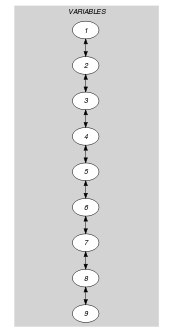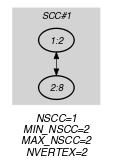(a) (b)
Automaton

Figures 5.173.25.173.45.173.6 and 5.173.8 depict the different automata associated with the $\mathrm{𝚐𝚛𝚘𝚞𝚙}_\mathrm{𝚜𝚔𝚒𝚙}_\mathrm{𝚒𝚜𝚘𝚕𝚊𝚝𝚎𝚍}_\mathrm{𝚒𝚝𝚎𝚖}$ constraint. For the automata that respectively compute $\mathrm{𝙽𝙶𝚁𝙾𝚄𝙿}$, $\mathrm{𝙼𝙸𝙽}_\mathrm{𝚂𝙸𝚉𝙴}$, $\mathrm{𝙼𝙰𝚇}_\mathrm{𝚂𝙸𝚉𝙴}$ and $\mathrm{𝙽𝚅𝙰𝙻}$ we have a 0-1 signature variable ${𝚂}_{i}$ for each variable ${\mathrm{𝚅𝙰𝚁}}_{i}$ of the collection $\mathrm{𝚅𝙰𝚁𝙸𝙰𝙱𝙻𝙴𝚂}$. The following signature constraint links ${\mathrm{𝚅𝙰𝚁}}_{i}$ and ${𝚂}_{i}$: ${\mathrm{𝚅𝙰𝚁}}_{i}\in \mathrm{𝚅𝙰𝙻𝚄𝙴𝚂}⇔{𝚂}_{i}$.

##### Figure 5.173.2. Automaton for the $\mathrm{𝙽𝙶𝚁𝙾𝚄𝙿}$ argument of the $\mathrm{𝚐𝚛𝚘𝚞𝚙}_\mathrm{𝚜𝚔𝚒𝚙}_\mathrm{𝚒𝚜𝚘𝚕𝚊𝚝𝚎𝚍}_\mathrm{𝚒𝚝𝚎𝚖}$ constraint and its glue matrix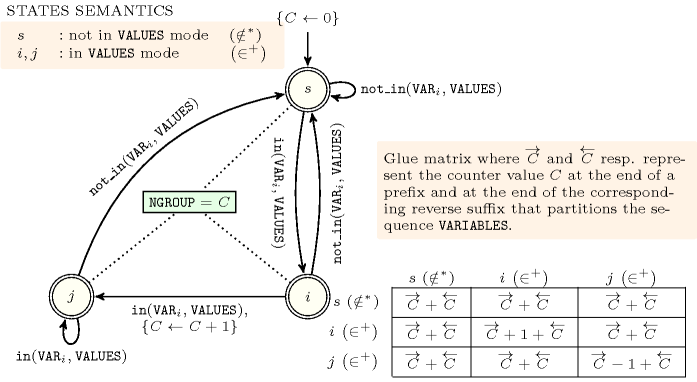##### Figure 5.173.3. Hypergraph of the reformulation corresponding to the automaton (with one counter) of the $\mathrm{𝙽𝙶𝚁𝙾𝚄𝙿}$ argument of the $\mathrm{𝚐𝚛𝚘𝚞𝚙}_\mathrm{𝚜𝚔𝚒𝚙}_\mathrm{𝚒𝚜𝚘𝚕𝚊𝚝𝚎𝚍}_\mathrm{𝚒𝚝𝚎𝚖}$ constraint (since all states of the automaton are accepting there is no restriction on the last variable ${Q}_{n}$)##### Figure 5.173.4. Automaton for the $\mathrm{𝙼𝙸𝙽}_\mathrm{𝚂𝙸𝚉𝙴}$ argument of the $\mathrm{𝚐𝚛𝚘𝚞𝚙}_\mathrm{𝚜𝚔𝚒𝚙}_\mathrm{𝚒𝚜𝚘𝚕𝚊𝚝𝚎𝚍}_\mathrm{𝚒𝚝𝚎𝚖}$ constraint and its glue matrix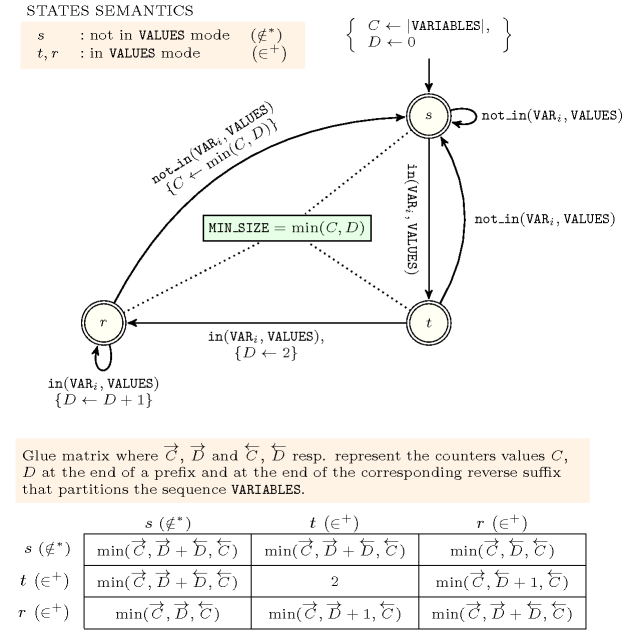##### Figure 5.173.5. Hypergraph of the reformulation corresponding to the automaton (with two counters) of the $\mathrm{𝙼𝙸𝙽}_\mathrm{𝚂𝙸𝚉𝙴}$ argument of the $\mathrm{𝚐𝚛𝚘𝚞𝚙}_\mathrm{𝚜𝚔𝚒𝚙}_\mathrm{𝚒𝚜𝚘𝚕𝚊𝚝𝚎𝚍}_\mathrm{𝚒𝚝𝚎𝚖}$ constraint where $𝙽$ stands for $|\mathrm{𝚅𝙰𝚁𝙸𝙰𝙱𝙻𝙴𝚂}|$ (since all states of the automaton are accepting there is no restriction on the last variable ${Q}_{n}$)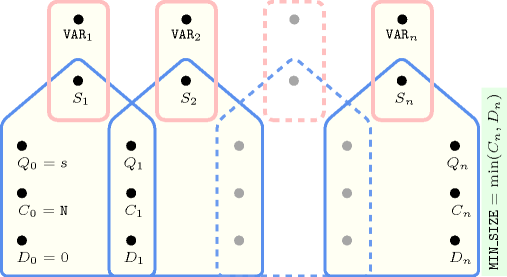##### Figure 5.173.6. Automaton for the $\mathrm{𝙼𝙰𝚇}_\mathrm{𝚂𝙸𝚉𝙴}$ argument of the $\mathrm{𝚐𝚛𝚘𝚞𝚙}_\mathrm{𝚜𝚔𝚒𝚙}_\mathrm{𝚒𝚜𝚘𝚕𝚊𝚝𝚎𝚍}_\mathrm{𝚒𝚝𝚎𝚖}$ constraint and its glue matrix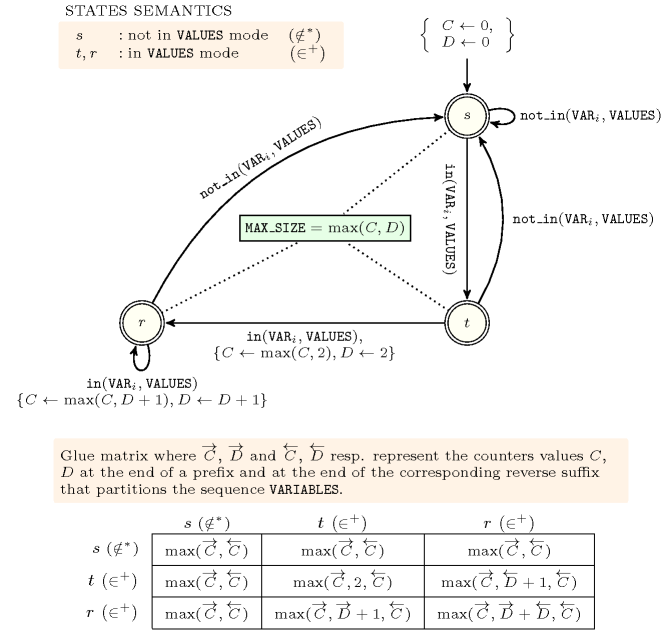##### Figure 5.173.7. Hypergraph of the reformulation corresponding to the automaton (with two counters) of the $\mathrm{𝙼𝙰𝚇}_\mathrm{𝚂𝙸𝚉𝙴}$ argument of the $\mathrm{𝚐𝚛𝚘𝚞𝚙}_\mathrm{𝚜𝚔𝚒𝚙}_\mathrm{𝚒𝚜𝚘𝚕𝚊𝚝𝚎𝚍}_\mathrm{𝚒𝚝𝚎𝚖}$ constraint (since all states of the automaton are accepting there is no restriction on the last variable ${Q}_{n}$)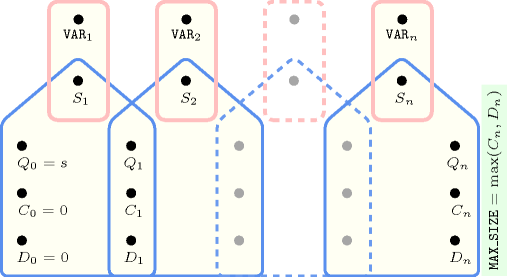##### Figure 5.173.8. Automaton for the $\mathrm{𝙽𝚅𝙰𝙻}$ argument of the $\mathrm{𝚐𝚛𝚘𝚞𝚙}_\mathrm{𝚜𝚔𝚒𝚙}_\mathrm{𝚒𝚜𝚘𝚕𝚊𝚝𝚎𝚍}_\mathrm{𝚒𝚝𝚎𝚖}$ constraint and its glue matrix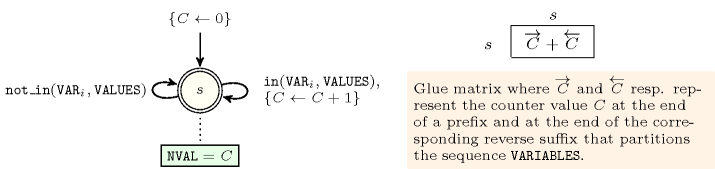##### Figure 5.173.9. Hypergraph of the reformulation corresponding to the automaton (with one counter) of the $\mathrm{𝙽𝚅𝙰𝙻}$ argument of the $\mathrm{𝚐𝚛𝚘𝚞𝚙}_\mathrm{𝚜𝚔𝚒𝚙}_\mathrm{𝚒𝚜𝚘𝚕𝚊𝚝𝚎𝚍}_\mathrm{𝚒𝚝𝚎𝚖}$ constraint